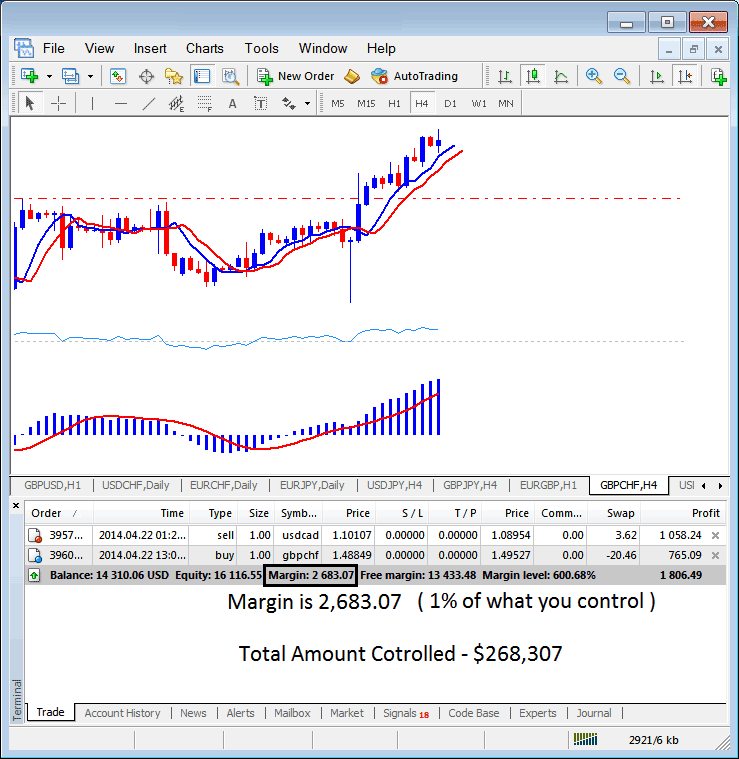## Free Indices Trading Margin Calculator and Used Indices Trading Margin Calculator

The indices margin calculation example explained and illustrated below, the set indices leverage ratio is 100:1, the indices margin which is 1% is \$2683.07, therefore the total amount controlled by the indices trader is: \$268,307 - this is because with this 100:1 leverage ratio, the indices trader has used little of their money and borrowed the rest using indices leverage, with this leverage ratio set at 100:1, the indices trader is using 1% of their capital, this 1% is \$2683.07, if 1% is \$2683.07 then 100% is \$268,307• If = 50:1 - Indices Leverage Ratio

Then indices margin requirement = 1/50 *100= 2%

if you have \$1,000,

1,000* 50 = \$50,000.

1,000 / 50,000 * 100= 2%

(Simplify - your capital is \$1,000 after leverage you control \$50,000 - \$1,000 is what percent of \$50,000 - it is 2% margin) that is your indices margin requirement

• If = 20:1 - Indices Leverage Ratio

Then the indices trading margin requirement = 1/20 *100= 5%

if you have \$1,000,

1,000* 20 = \$20,000.

1,000 / 20,000 * 100= 5%

(Simplify - your capital is \$1,000 after leverage you control \$20,000 - \$1,000 is what percent of \$20,000 - it is 5% margin) that is your indices trading margin requirement

• If = 10:1 - Indices Leverage Ratio

Then the indices margin requirement is = 1/10 *100= 10%

if you have \$1,000,

1,000* 10 = \$10,000.

1,000 / 10,000 * 100= 10%

(Simplify - your capital is \$1,000 after leverage you control \$10,000 - \$1,000 is what percent of \$10,000 - it is 10% margin) that is your indices trading margin requirement